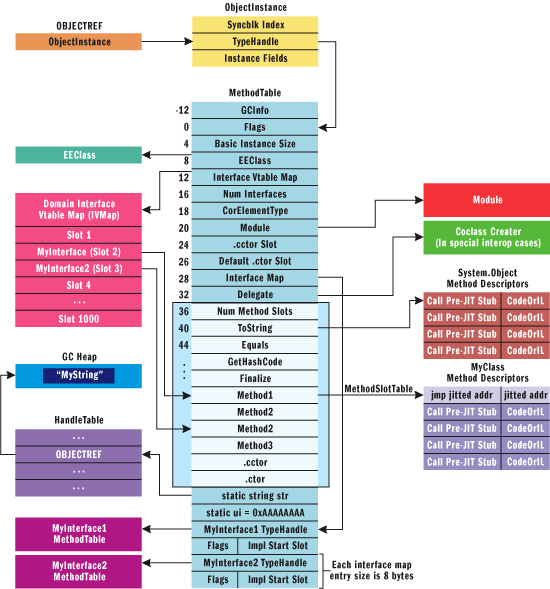# .NET学习笔记（五） ——类型设计（下）

———————————————————————————————————————————–

（接上篇）

##### 1. Int32里是类似下面的方法，可以发现实际是调用了Convert.ToInt16里的实现 并且是受保护的方法。内部也没有转换操作符 。
short   IConvertible.ToInt16 (IFormatProvider   provider)
{
return   Convert.ToInt16(this.m_value);
} 
##### 2. decimal里面也与这样的受保护的实现方法
short   IConvertible.ToInt16 (IFormatProvider   provider)
{
return   Convert.ToInt16(this);
} 

public   static   short   ToInt16(decimal   value)
{
int   num   =   ToInt32(value);
if   ((num   <   -32768)   ¦ ¦   (num   >   0x7fff))
{
throw   new   OverflowException(Environment.GetResourceString ("Overflow_Int16"));
}
return   (short)    num;
} 

3. 在看下Convert中的实现，实际他是调用decimal内部实现

public   static   short   ToInt16(decimal   value)
{
return   decimal.ToInt16 (decimal.Round (value,   0));
}  
##### 4. 而对于那些内部没有实现ToXXX方法的，是在Convert中实现的。
public   static   short   ToInt16(int   value)
{
if   ((value   <   -32768)   ¦ ¦   (value   >   0x7fff))
{
throw   new   OverflowException(Environment.GetResourceString ("Overflow_Int16"));
}
return   (short)    value;
} 
1. 看完上面基本明白了， 对IConvertible接口方法的实现，都是调用Convert类中的方法。
2. 基元类型 的转换的实现基本都在Convert里面，而内部没有实现，所以要通过Convert实现转换。
3. 对于内部实现了的ToXXX()方法的（比如 Decimal），我们可以直接调用此静态方法，也可以通过Convert的方法（其实他也是调用内部实现了的ToXXX()方法）
4. 对于采用 强制类型转换，基元类型会自动产生IL指令，而其他类型则调用转换操作符方法。

# 五 方法的参数

### 1 引用参数

CLR允许我们通过关键字（out,ref）来定义参数通过引用方式传递（这里引用方式传递叫引用参数，他和引用类型参数是不同的）。这 两个关键字会告诉编译器，产生额外的元数据来表示参数是按照引用方式传递。两个关键字的区别在与out的参数在使用前可以不被初始化 ，并且被调用方法不能直接读取参数的值，它必须在返回前给；而ref要求参数在使用前被初始化，被调用的方法可以任意读取该参数。

out方法：

class ex1
{
static void Main()
{
Int32 x;
SetVal (x);              //x不需要被初始化
Console.WriteLine(x);   //显示“10”

}
static void SetVal(out Int32 v)
{
v =10;                 //此方法必须初始化v
}
}

ref方法:

class ex2
{
static void Main()
{
Int32 x = 5;
Console.WriteLine (x);   //显示 “15”

}
{
v+=10;                 //可以直接初始化v
}
}

static void add(Point p){.....}

static void add(ref Point p){.....} //但这2个关键的不能同时存在

class cc
{
public Int32 val;
}

class ex
{
static void Main()
{
cc c ;
change(out c);        //error CS1503: 参数“1” : 无法从“out cc”转换为“out object”
Console.WriteLine(c.val);

}

static void change(out Object o)
{
o = new String('x',100);
}

class PassingRefByVal
{
static void Change(int[] pArray)
{
pArray = 888;  // This change affects the original element.
pArray = new int {-3, -1, -2, -3, -4};   // This change is local.
System.Console.WriteLine("Inside the method, the first element is: {0}", pArray);
}

static void Main()
{
int[] arr = {1, 4, 5};
System.Console.WriteLine("Inside Main, before calling the method, the first element is: {0}", arr );

Change(arr);
System.Console.WriteLine("Inside Main, after calling the method, the first element is: {0}", arr );
}
}


Inside Main, before calling the method, the first element is: 1

Inside the method, the first element is: -3

Inside Main, after calling the method, the first element is: 888


class PassingRefByRef
{
static void Change(ref int[] pArray)
{
// Both of the following changes will affect the original variables:
pArray = 888;
pArray = new int {-3, -1, -2, -3, -4};
System.Console.WriteLine("Inside the method, the first element is: {0}", pArray);
}

static void Main()
{
int[] arr = {1, 4, 5};
System.Console.WriteLine("Inside Main, before calling the method, the first element is: {0}", arr);

Change(ref arr);
System.Console.WriteLine("Inside Main, after calling the method, the first element is: {0}", arr);
}
}

Inside Main, before calling the method, the first element is: 1

Inside the method, the first element is: -3

Inside Main, after calling the method, the first element is: -3

### 2 可变数目参数

static Int32 Add(pararms Int32[] values)
{
Int32 sum = 0;
for (Int32 x = 0; x<values.Length;x++)
{
sum += values[x];
}
return sum;
}

### 3 虚方法# 六  属性

### 1 无参属性

class ex
{
int n;
public ex()
{
n = 1;
}

public void SetVal (int n)
{
if(n<0)
Console.WriteLine("n要大于等于0");
else
this.n = n;
}

public int GetVal()
{
return this.n;
}

}

class examlpe
{
static void Main()
{
ex o = new ex();
Console.WriteLine(o.GetVal());
o.SetVal(10);
Console.WriteLine(o.GetVal());
o.SetVal(-10);
Console.WriteLine(o.GetVal());

}
}

1. 要写一些额外的代码来访问。
2. 只能通过方法名来访问字段，没有直接访问直观。

CLR提供的属性，来缓解了第一个问题和完全解决了第二个问题。修改后的代码如下。

class ex
{
int _n;             //定义的私有字段用'_'避免命名冲突
public ex()
{
_n = 1;
}

public int n
{
get{ return _n; }
set
{
if(value<0)
Console.WriteLine("n需要大于等于0");
else
_n = value;
}
}
}

class examlpe
{
static void Main()
{
ex o = new ex();
Console.WriteLine(o.n);
o.n = 10;
Console.WriteLine(o.n);
o.n =- 10;
Console.WriteLine(o.n);

}
}

.method public hidebysig specialname instance int32  get_n() cil managed

.method public hidebysig specialname instance void set_n(int32 'value') cil managed

### 2 含参属性

class BitArray
{
//保存位的私有字节数组
private Byte[] byteArray;
private Int32 numBits;

//构造函数分配字节数组，所有位置0
piblic BitArray(Int32 numBits)
{
if (numBits<=0)
throw new ArgumentOutRangeRxception("numBits must be>0");
this.numBits = numBits;
byteArray = new Byte[(numBits + 7)/8];
}

//索引器
public Boolean this[Int32 bitPos]
{
get
{
//检查访问数组位数的有效性
if((bitPos < 0) || (bitPos >= numBits))
throw new IndexOutOfRangeException();
//返回指定索引上的位状态
return (byteArray[bitPos / 8] & (1 << (bitPos % 8))) != 0;
}
set
{
if ((bitPos < 0) || (bitPos >= numBits))
throw new IndexOutOfRangeException();

if(value)
byteArray[bitPos / 8] = (Byte)(byteArray[bitPos / 8] | (1 << (bitPos% 8)));
else
byteArray[bitPos / 8] = (Byte)(byteArray[bitPos / 8] & ~(1 << (bitPos% 8)));

}
}

}

class examlpe
{
static void Main()
{
//分配一个含14个位的BitArray
BitArray ba = new BitArray(14);

//调用set访问器
for(Int32 x = 0;x < 14;x++)
ba[x] = (x % 2 ==0);

//调用get访问器
for(Int32 x = 0;x < 14;x++)
Console.WriteLine("Bit" + x + "is " + (ba[x] ? "On" : "Off"));

}
}

Public class BitArray
{
[System.Runtime.ComplierServices.IndexerName("Bit")]
Public Boolen this[Int32 bitPos]
}
Console.WriteLine(ba);
Console.WriteLine(ba.Bit);